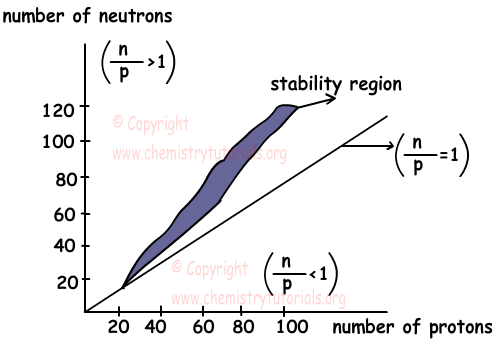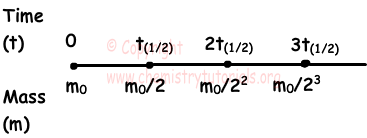EN | ES | DE | NL | RU

## Nuclear Chemistry (Radioactivity) Cheat Sheet

Nuclear chemistry or radioactivity deals with changes in the structure of nucleus. There are protons and neutrons in nucleus of atoms. Protons are positively charged and neutrons are neutral particles. Since same particles repel each other, protons repel each other. Neutrons placed between protons and decrease repulsion force between protons. Ratio between number of protons and neutrons in nucleus shows whether atom is stable or unstable. If;

n0/p+≈1 then atom is stable

n0/p+<1 or n0/p+>1,5 nucleus of atoms are unstable and we call these atoms radioactive elements.

Unstable atoms do some nuclear reactions like radiation or decay and become stable atoms. We can explain radioactivity under two titles, natural nuclear reactions and artificial nuclear reactions. In natural reactions, unstable atoms do radiation and become stable atoms. However, in artificial reactions, unstable atoms can be turn in to stable atoms artificially.

Differences between Chemical Reactions and Nuclear Reactions: (C.R: chemical Reaction, N.R: Nuclear Reaction)

• In chemical reactions, atoms are organized by breaking chemical bonds and forming new ones. On the contrary, elements or isotopes of elements can turn into other elements in nuclear reactions.
• In C.R only valence electrons play role in breaking and forming bonds, however in N.R protons, neutrons and electrons play role.
• IN C.R. types of atoms are conserved but in N.R, types of atoms can be changed.
• Mass is conserved in C.R. but mass is not conserved in N.R.

Graph given below shows stability of nucleus;Alpha (α) particles can be called Helium-4 nuclei (24He+2). After alpha decay, atomic number of nucleus decreases by 2 and mass number decreases by 4 and number of neutrons also decreases by 2.

Beta radiation is formed during conversion of one neutron to one proton. Particle produced after this process is electron. We show it in nuclear reactions with Greek letter "β-"

01n → 11p + -10e(β-)

It is also called, beta positive decay. It is denoted by +10e or β+. Positron decay is conversion of one proton to one neutron.

11p → 01n + +10e

Gamma radiations are short wave length electromagnetic waves. Gamma decays occur after other radiations to emit excess energy of nucleus to become stable. Gamma radiation is shown with "γ".

5. Electron Capture:

Some of nuclei capture one electron on inner shell of it. This electron convert one proton to one neutron in nucleus.

11p + -10e → 01n

1. Nuclear Fission:

Nuclear fission is a nuclear reaction in which nucleus of atom split into smaller particles. Nucleus having mass number larger than 200 does neutron decay and split into elements having smaller mass numbers.

2. Nuclear Fusion:

More than one nucleus, having small atomic masses, are combined to form heavier new nucleus. Nuclear fusion is also exothermic reactions and energy released in these reactions are larger than energy released in fission reactions. On the contrary, there must be large amount of energy to start fusion reactions.In hydrogen bomb we see fusion reactions.

Half Life: Unstable nucleus does radioactive decays and decrease its mass. Half time is time required for half of mass of radioactive matter to decay. It is depends on types of matter or n/p ratio. If initial mass of matter is m0, after t time it has mass m, and if half life of matter is t(1/2);

when t=t(1/2) m=m0/2

Picture given below shows amount of mass as the time passes;After first half time, mass decreases to m0/2, after second half time it decreases half of its previous value. If we write it as equation we get;

m=m0/2n

Where "n" is the number of half life,

n=t/t(1/2)

Rate of Decay:

Rate of decay is number of disintegrated nucleus in unit time. Rate of decay depends on half life and mass of matter.

• Rate of decay is inversely proportional to half life of matter. If masses of two matters are equal than matter having smaller half life has higher rate of decay.
• Rate of decay is directly proportional to mass of radioactive matter.
• Since mass of matter decreases in decay process, rate of decay also decreases with time.

Relation between half life and rate of decay is;

k=0,693/t(1/2)

where k is rate of decay and t(1/2) is half life.

The Original Author: